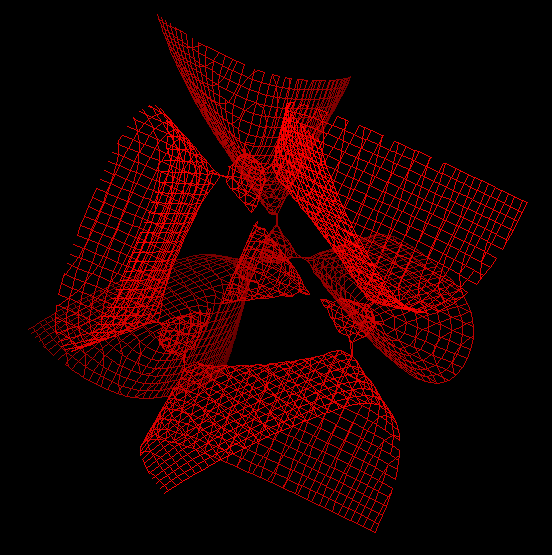# Kummer Quartic

A Kummer surface is any one of a one parameter family of algebraic surfaces defined by the polynomial equation of degree four:

```(x^2 + y^2 + z^2 - b^2)^2 - λ*p*q*r*s = 0.
Here b is any real number.

λ = (3*b^2 - 1.0)/(3 - b^2)
p = 1 - z - Sqrt*x
q = 1 - z + Sqrt*x
r = 1 + z + Sqrt*y
s = 1 + z - Sqrt*y```

kummer.gcf

The formula fully expanded looks like this: -(((-1 + 3*b^2)*(1 - Sqrt*x - z)*(1 + Sqrt*x - z)*(1 - Sqrt*y + z)*(1 + Sqrt*y + z))/(3 - b^2)) + (-b^2 + x^2 + y^2 + z^2)^2

The family was described originally by Ernst Eduard Kummer In 1864.

A Kummer surface has sixteen double points, the maximum possible for a surface of degree four in three-dimensional space. For the default case b = 1.3, all these double points are real and they appear in the visualization as the vertices of five tetrahedra.a wire-frame view, showing its tetrahedron symmetry.

Mathematica file

If you have a question, put \$5 at patreon and message me.